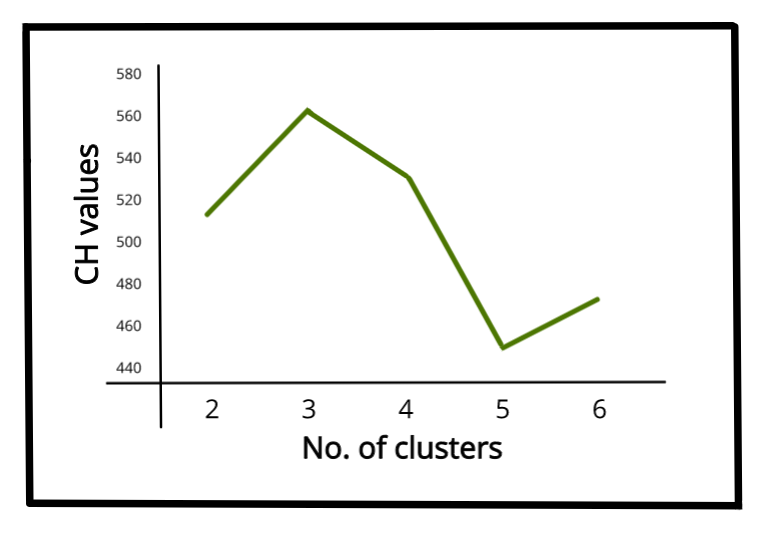# Calinski-Harabasz Index – Cluster Validity indices | Set 3

• Last Updated : 25 Apr, 2022

Prerequisites: Cluster Validity Index

Clustering validation has been recognized as one of the important factors essential to the success of clustering algorithms. How to effectively and efficiently assess the clustering results of clustering algorithms is the key to the problem. Generally, cluster validity measures are categorized into 3 classes (Internal cluster validation, External cluster validation and Relative cluster validation).

In this article, we focus on an internal cluster validation index i.e. Calinski-Harabasz Index.

Calinski-Harabasz Index:

Calinski-Harabasz (CH) Index (introduced by Calinski and Harabasz in 1974) can be used to evaluate the model when ground truth labels are not known where the validation of how well the clustering has been done is made using quantities and features inherent to the dataset. The CH Index (also known as Variance ratio criterion) is a measure of how similar an object is to its own cluster (cohesion) compared to other clusters (separation). Here cohesion is estimated based on the distances from the data points in a cluster to its cluster centroid and separation is based on the distance of the cluster centroids from the global centroid. CH index has a form of (a . Separation)/(b . Cohesion) , where a and b are weights.

Calculation of Calinski-Harabasz Index:

The CH index for K number of clusters on a dataset D =[ d1 , d2 , d3 , … dN ] is defined as,where, nk  and ck  are the no. of points and centroid of the kth cluster respectively, c is the global centroid, N is the total no. of data points.

Higher value of CH index means the clusters are dense and well separated, although there is no “acceptable” cut-off value. We need to choose that solution which gives a peak or at least an abrupt elbow on the line plot of CH indices. On the other hand, if the line is smooth (horizontal or ascending or descending) then there is no such reason to prefer one solution over others.Line-plot of CH values vs No. of clusters for IRIS dataset

Below is the Python implementation of the above CH index using the sklearn library :

## python3

 from sklearn import datasets from sklearn.cluster import KMeans from sklearn import metrics from sklearn.metrics import pairwise_distances import numpy as np   # loading the dataset X, y = datasets.load_iris(return_X_y=True)   # K-Means kmeans = KMeans(n_clusters=3, random_state=1).fit(X)   # we store the cluster labels labels = kmeans.labels_   print(metrics.calinski_harabasz_score(X, labels))

Output:

561.62

References:

My Personal Notes arrow_drop_up
Recommended Articles
Page :